# A Facial Expression Recognition Model Based on Texture and Shape Features

A Facial Expression Recognition Model Based on Texture and Shape Features
College of Data Science and Software Engineering, Baoding University, Baoding 071000, China

Corresponding Author Email:
anlei@bdu.edu.cn
Page:
627-632
|
DOI:
https://doi.org/10.18280/ts.370411
31 March 2020
|
Accepted:
27 June 2020
|
Published:
10 October 2020
| Citation

OPEN ACCESS

Abstract:

With the development of computer vision, facial expression recognition has become a research hotspot. To further improve the accuracy of facial expression recognition, this paper probes deep into image segmentation, feature extraction, and facial expression classification. Firstly, the convolution neural network (CNN) was adopted to accurately separate the salient regions from the face image. Next, the Gaussian Markov random field (GMRF) model was improved to enhance the ability of texture features to represent image information, and a novel feature extraction algorithm called specific angle abundance entropy (SAAE) was designed to improve the representation ability of shape features. After that, the texture features were combined with shape features, and trained and classified by the support vector machine (SVM) classifier. Finally, the proposed method was compared with common methods of facial expression recognition on a standard facial expression database. The results show that our method can greatly improve the accuracy of facial expression recognition.

Keywords:

Facial expression recognition, texture features, shape features, Gaussian Markov random field (GMRF) model, support vector machine (SVM) classifier

1. Introduction

Facial expression directly reflects the real-time emotions of humans, offering a more direct and authentic way to exchange information than language. In recent years, great advancement has been made in facial expression recognition, with the continuous progress in computer vision and artificial intelligence (AI). Facial expression recognition is increasingly applied to such fields as education, medical, business, and transport. Despite the wide application, there are many problems in facial expression recognition. The most prominent problem is the low accuracy, which limits the further application of facial expression recognition.

The accuracy of facial expression recognition is greatly affected by the imaging environment and object. Any variation in the imaging environment will bring a change to the image background. As for the object, the hair has a certain occlusion effect on the face area, and the clothes determine the color diversity of the image, affecting the extraction of texture. Therefore, face images must be preprocessed, and the processing effect directly bears on the result on facial expression recognition. In addition, facial expression changes with the actions of facial muscles. To capture the subtle changes of facial expression, more details of facial changes must be extracted.

In facial expression recognition, the most important steps are feature extraction and feature fusion. The purpose of feature extraction is to get the mathematical data that can effectively describe image information, and improve the accuracy of image classification. The ability of the extracted features to represent image information determines the final effect of facial expression recognition. Feature fusion is to merge the various features extracted from the image. In this paper, the accuracy of facial expression recognition is improved by processing the texture and shape features of the image.

2. Literature Review

Facial expression recognition has long been a research hotspot. Based on abundant data and experimental results, Ekman and Friesen  divided facial expressions into six widely recognized categories, namely, happiness, disgust, sadness, fear, surprise, and anger, and sums up the theories on expression recognition, laying a solid basis for the research of facial expression classification. Pu et al.  attributed the variation in facial expressions to the actions of facial muscles triggered by the nerve impulses at emotional changes, suggested that the changing facial expressions will cause facial features (e.g. eyes, mouth, and nose) to move and deform, and proposed an estimation method for facial expression by extracting the optical flow from the facial texture features.

Uçar et al.  designed a radial basis function (RBF) network, capable of mining the correlation between the motion patterns of facial features and human facial expressions, and applied the trained network to analyze facial expressions. Lu et al.  transformed face images into eigenvectors for two-dimensional (2D) discrete cosine transform, and treated single-layer feedforward neural network as the classifier, aiming to improve the generalization and classification of facial expressions. Lee and Ro  created a novel feature of facial expressions, which encodes the amplitude and direction of the edges of the region of interest (ROI) to express different facial expression features, and demonstrated that the feature is highly robust to noise and illumination changes.

To recognize facial expressions in videos, Lopes et al.  proposed a three-dimensional (3D) convolutional neural network (CNN), consisting of a 3D Inception-ResNet layer and a long short-term memory (LSTM) unit, and experimentally demonstrated the effectiveness of the network. To enhance the resistance of local edge-based expression features to position change and noise, Chen and Hu  presented a local prominent direction pattern algorithm that selects the effective edge and encodes it by searching the local neighborhood of the image, thereby reducing the impact of position change on the effectiveness of features. Gu et al.  improved the original Gabor features to promote the poor performance of global features and reduce the redundancy of feature data, and developed a feature combination method to extract expression features.

Wang et al.  studied the relationship between facial expression changes and facial muscles, and put forward a facial expression modeling method based on interval algebraic Bayesian network, which can simultaneously extract spatial and temporal sequence features of the face, and speed up training and classification. Zhao and Zhang  improved the local discriminant analysis (LDA) for facial expression recognition and classification, and manifested the strong robustness of the improved method. Xu et al.  developed a detail aware migration network for facial expression recognition. The network can extract the features of receptive field at various scales, reduce the loss of high-level features of the image, and reduce the number of network parameters and training time. Sultana et al.  improved the accuracy of facial expression recognition by the local binary pattern (LBP) based on feature points.

3. CNN-Based Face Region Segmentation

Image preprocessing  is the first step of image processing, and a key link in facial expression recognition. The purpose of preprocessing face image is to extract the features of facial expressions from the region of interest (ROI) . The quality of the extracted features hinges on the preprocessing effect, exerting a huge impact on the training effect and the accuracy of facial expression recognition.

In the facial expression dataset, the face images have different illuminations and backgrounds. Besides, the face and lip regions vary from image to image, and the hairstyle and clothes change from object to object. As a result, it is of great necessity to preprocess face images.

3.1 Face region separation

Face image preprocessing includes two steps: face region separation, and face region segmentation. The former aims to separate the face region from the original image by eliminating the interference of hair, clothes, and other factors, and the latter aims to divide the segmented face region into different subblocks.

In the facial expression dataset, each face image has a unique background, and each object has his/her unique hairstyle and face size. These factors must be considered during the feature extraction from face images.

## 1.png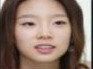Figure 1. An example of face image from facial expression dataset

As shown in Figure 1, the woman is making a smile expression. The shape and position of her hair, together with the proportion of hair in the image area, will greatly influence the extraction of texture features: the hair region will increase the intra-class difference of feature information, and lower the accuracy of facial expression recognition. To make accurate recognition of facial expressions, it is very important to separate the face region from the original face image, that is, remove the hair, clothes, and background. After the separation, the whole image is occupied by the face region (Figure 2), eliminating the impacts of face position and face proportion on feature extraction.

## 2.png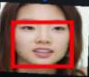Figure 2. The image after face region separation

3.2 Face region segmentation

On the face image, the variation of facial expression is very subtle. By separating the face region from the image, many negative factors on facial expression recognition are excluded. But face image segmentation alone is not enough. If features are extracted from the entire face region, it will be difficult to prevent the loss of some feature information, and distinguish between different features.

Therefore, this paper proposes a method for face region segmentation. After being separated from the dataset, the face region was divided into a number of subblocks. Depending on the actual image size of the dataset, each face region can be divided into 9, 16, 25, 36, 49, or 64 subblocks. After the segmentation, each subblock can be compared to acquire the details of expression changes, and better recognize the facial expression. This is obviously more refined than the recognition based on the entire face region.

Since the position and shape of facial features change with facial expressions, the variation of facial features was taken as the basis of facial expression. Because of the physiological structure, the ears and nose will not change much when humans change facial expressions. Therefore, these facial organs were neglected in feature extraction. During the change of facial expression, the eyebrows could either raise or frown, which contribute little to the discrimination between different facial expressions. Considering the individual difference in eyebrow shape, the eyebrows were also excluded from feature extraction. Despite having richer changes, the eyes only account for a small proportion in the face region, failing to provide sufficient information to distinguish between facial expressions. The eyebrows and eyes is illustrated in Figure 3.

## 3.png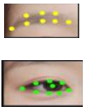Figure 3. The recognition of eyebrows and eyes

Through the above analysis, the mouth was chosen as a factor to recognize facial expressions, for its diverse changes between facial expression: the mouth opens wide in the surprise expression, goes up in the happiness expression, and droops in the sadness expression. Moreover, the mouth, covering a larger area than the eyes, makes it easier to differentiate between different classes of shape features.

To extract the shape features of different facial expressions, the mouth region was segmented by the full convolutional network (FCN) . In the traditional CNN, the input images must be consistent in size, due to the presence of the fully connected layer. The FCN eliminates the fully connected layer, allowing the input images to have random size. Figure 4 provides an example of mouth region extracted by the FCN.

## 4.png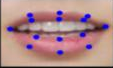Figure 4. An example of mouth region segmentation

4. Design of Feature Fusion Algorithm

The features obtained by a single feature extraction algorithm cannot represent the information of the entire face image. To solve the problem, this paper designs an improved feature fusion algorithm based on Gaussian Markov random field (GMRF).

4.1 LBP-based improved GMRF model

The difficulty of facial expression recognition lies in the extraction of the details on facial expressions. The original GMRF model is not sufficiently refined to achieve this purpose. Therefore, this paper improves the GMRF model by fusing LBPs.

In the GMRF model, the number of pixels in the neighborhood of the central pixel differs with the orders. The LBP features require eight (or multiples of eight) neighborhood pixels. If the feature order of GMRF is two, there are eight pixels in the neighborhood. If the order is five, there are 24 pixels in the neighborhood. The number of neighborhood pixels under the two orders is the minimum number of LBP coding and integer times. Therefore, the GMRF features of these two orders were selected as the objects of improvement.

In the second-order GMRF, there are eight pixels in the neighborhood of the central pixel, and the traditional LBPs are encoded in the order of the basic method. The traditional LBP value of neighborhood centering on pixel v can be expressed as:

\begin{aligned} L B P_{S, T}\left(x_{c}, y_{c}\right)=& \sum_{S=0}^{7} V\left(g_{S}-g_{N}\right) 2^{S} V(\mathrm{x}) \\ &=\left\{\begin{array}{ll}1 & x \geq 0 \\ 0 & x<0\end{array}\right.\end{aligned}     (1)

In the fifth-order GMRF, there are 24 pixels in the neighborhood of the central point, which is encoded clockwise from the top left corner of the layer. The LBP is encoded according to this coding sequence, producing three LBP values. The traditional LBP value of neighborhood centering on pixel v can be expressed as:

$V_{L B P(S, T)}^{\prime}(v)=\sum_{S=0}^{7} V\left(g_{S}-g_{v}\right) 2^{S} R=1$

$V_{L B P(S, T)}^{\prime \prime}(v)=\sum_{S=8}^{15} V\left(g_{S}-g_{v}\right) 2^{S} R=2$      (2)

$V_{L B P(S, T)}^{\prime \prime \prime}(v)=\sum_{S=16}^{23} V\left(g_{S}-g_{v}\right) 2^{S} R=2$

The main idea of the improved GMRF algorithm is to fuse the LBPs, and estimate the eigenvectors by the GMRF. It can be implemented in the following steps:

Step 1. Fusion between LBP and GMRF

Image feature fusion is the primary method to amplify the number of features, and enhance the effectiveness of information in image processing. Through the fusion, multiple features can be used simultaneously to represent the information contained in the image. The fused features facilitate the computer analysis and interpretation of image information. The original central neighborhood of the GMRF can be fused with the LBPs as a linear equation. The linear equations for the second- and fifth-order GMRFs fused with LBPs can be respectively expressed as:

$f(v)=\sum_{j \in N} \rho_{j}^{*}[f(v+j)+f(v-j)]+\rho_{j+1}^{*} \cdot V_{L B P}$$\quad+\rho_{j+2}^{*} \cdot V_{L B P}+\varepsilon(v)$      (3)

\begin{aligned} f(v)=\sum_{j \in N} \rho_{j}^{*}[f(v&+j)+f(v-j)]+\rho_{j+1}^{*} \cdot V_{L B P}^{\prime} \\ &+\rho_{j+2}^{*} \cdot V_{L B P}^{\prime \prime}+\rho_{j+3}^{*} \cdot V_{L B P}^{\prime \prime \prime}+\rho_{j+4}^{*} \\ & \cdot V_{G B P}^{\prime}+\rho_{j+5}^{*} \cdot V_{G B P}^{\prime \prime}+\rho_{j+6}^{*} \cdot V_{G B P}^{\prime \prime \prime} \\ &+\varepsilon(v) \end{aligned}     (4)

where, N is the neighborhood of point v; j is the neighborhood radius; $\rho$ is the coefficient of GMRF; $V_{L B P}$ is the LBP value of the neighborhood; VGBP is the improved LBP value; $\rho_{j}^{*}$ is the new coefficient of the improved GMRF model.

Step 2. Model construction

For each neighborhood central point in the image, N×N equations about f(v) can be obtained. The improved GMRF model can be defined as:

$y=Q^{T} \rho^{*}+\varepsilon$     (5)

where, ρ* is the eigenvector to be estimated.

Step 3. Eigenvector estimation

The parameter ρ* can be estimated by the least squares criterion:

$\hat{\rho}^{*}=\left(\sum_{V} Q_{V} Q_{V}^{T}\right)^{-1}\left(\sum_{V} Q_{V} f_{V}\right)$       (6)

4.2 Shape feature extraction by specific angle abundance entropy (SAAE)

When the facial expression changes, the mouth shape in the face region will also change, which inspires the study of facial expression recognition from organ shape. The SAAE  is a shape feature extraction method, capable of depicting the mouth shapes under different facial expressions.

## 5.png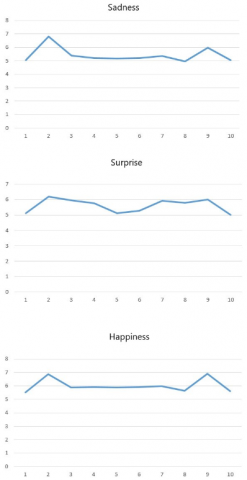Figure 5. The SAAE curves of the mouth in 10 dimensions under different facial expressions

The SAAE introduces entropy, a statistical concept, into feature expression. The abundance entropy of a specific angle is a shape feature. The SAAE feature describes the uncertainty of the edge contour of each subregion, after the mouth is divided by the centroid point based on the angle. Firstly, the transverse distance between the edge point and the centroid of each subregion was calculated. Then, the entropy of the distance in each subregion was computed. The smaller the entropy, the closer the edge to the line. The entropy of the transverse distance between the edge point and the centroid of each subregion constitutes the edge shape feature of the mouth.

To calculate the abundance entropy of the mouth at each angle, it is necessary to solve the coordinates ( $O_{x}, O_{y}$ ) of the centroid O of the mouth. Let $P\left(P_{x}, P_{y}\right)$ be the coordinates of each non-zero edge pixel. Then, the angle $\alpha$ between the pixel and the sine of the centroid can be calculated by:

$\hat{\alpha}=\tan ^{-1}\left(P_{y}-O_{y}\right) /\left(P_{x}-O_{x}\right) \times 180 / \pi$     (7)

$\left\{\begin{array}{lr}\alpha=\hat{\alpha} & \hat{\alpha}<0 \\ \alpha=360+\hat{\alpha} \text { else }\end{array}\right.$     (8)

where, $\hat{\alpha}$  is multiplied by 180/π to convert the arccosine into degrees. After the conversion, the value of $\alpha$ falls in the range of (-180, 180).

The abundance shape entropy can be calculated for each subregion of the mouth:

$H_{E_{C}}=-\sum_{(i, j) \in E_{C}} P_{i, j} \log _{a} P_{i, j}$     (9)

$P_{i j}=d_{i j} / \sum_{(i, j) \in E_{C}} d_{i j}=\left|j-O_{x}\right| / \sum_{(i, j) \in E_{C}}\left|j-O_{x}\right|$     (10)

where, $d_{i j}$ is the horizontal axis distance between pixel (i, j) on a specific angle contour and the centroid of the whole contour; $H_{E_{C}}$  is the entropy of contour $E_{C}$; P is the length distribution probability at pixel (i, j).

To sum up, the SAAE method divides the mouth region into different subregions by angle. The transverse distance between the edge pixel and the centroid is calculated for each subregion, and the entropy of each subregion is taken as a feature dimension of the SAAE. Therefore, the eigenvector dimensions of the abundance entropy of the mouth angle are different under different angles.

Here, the mouth region of a face is divided into 1-15 dimensions, and the SAAE shape features are extracted under different dimensions. Figure 5 shows the SAAE curves of the 10 dimensions under different facial expressions.

5. Experiments and Result Analysis

The effectiveness of the proposed improved GMRF and SAAE feature extraction methods were verified through experiments on the Japanese Female Facial Expression (JAFFE) Database. The database provides face images on many people with seven facial expressions: anger, disgust, fear, happiness, neutral, sadness, and surprise. Each person has two or four images of each facial expression.

Two cases were designed for the experiments: First, the features were obtained from the entire face region, before being trained and classified by support vector machine (SVM); Second, the features were obtained from different subregions of the face region before being trained and classified by the SVM. In both cases, the results of the improved GMRF was compared with those of the original GMRF.

Table 1. The accuracies of facial expression recognition by the second- and fifth- order GMRFs

 Number of subregions Second-order GMRF Fifth-order GMRF 1 0.3018 0.3877 9 0.5382 0.6338 16 0.5941 0.6229 25 0.7243 0.7102 36 0.6815 0.6815 49 0.7243 0.7102 64 0.7102 0.5672

Table 2. The accuracies of facial expression recognition by the second- and fifth- order improved GMRFs

 Number of subregions Second-order improved GMRF Fifth-order improved GMRF 1 0.3621 0.4635 9 0.5382 0.6667 16 0.6527 0.6938 25 0.7531 0.7386 36 0.7386 0.7927 49 0.7927 0.8113 64 0.7243 0.6522

The feature dimensions of the second-order GMRF fused with LBPs and fifth-order GMRF fused with LBPs are 6 and 18, respectively. Tables 1 and 2 compare the accuracies of facial expression recognition by the second- and fifth- order GMRFs with those achieved by the improved GMRFs on the same orders.

As shown in Tables 1 and 2, the improved GMRFs achieved better recognition accuracy than GMRFs in most cases of using the entire face region. With the growing number of subregions (i.e. the details of facial expressions), the recognition accuracy of each method firstly increased. However, the recognition accuracy started to decrease, after the number reached 64. This is because each subregion has too few pixels, when the face region is divided into too many subregions. To extract the details of the face image, special attention should be paid to the relationship between image size and the number of subregions.

## 6.pngFigure 6. The comparison between second-order GMRF and improved GMRFs with different subregion combinations

To further verify the effectiveness of feature fusion, the LBPs and GMRF features extracted with different number of subregions were combined, and classified by the SVM. Figures 6 and 7 compare the recognition accuracies achieved by the GMRFs and improved GMRFs of the second and fifth orders, at different subregion combinations.

As shown in Figures 6 and 7, the fusion of features extracted from different subregions promotes the recognition accuracy of facial expressions. The greater the number of subregions, the higher the recognition accuracy. The reason is that more image details are obtained from the various subregions. However, the recognition accuracy plunged for the combination between 9 and 64 subregions, for the 64 subregions each contain too few pixels. The limited number of pixels is sufficient to characterize the facial expressions, pushing up misclassification.

## 7.png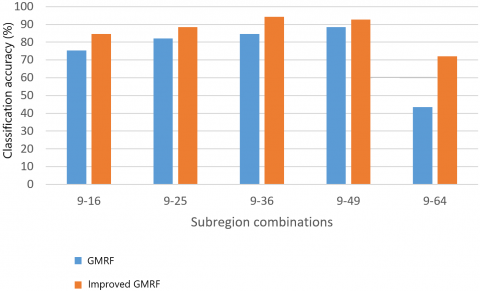Figure 7. The comparison between fifth-order GMRF and improved GMRFs with different subregion combinations

Texture features and shape features describe the facial expressions in the face image from different angles. To further improve the effectiveness and comprehensiveness of feature information, the GMRF features fused with LBPs were combined with mouth SAAE features, and then trained and classified by the SVM. The recognition accuracies were compared with different subregion combinations.

Table 3. The recognition accuracies of improved GMRF combined with SAAE features

 Subregion combination Second-order GMRF Fifth-order GMRF 9-16 0.8556 0.8992 9-25 0.8992 0.8992 9-36 0.9297 0.9421 9-49 0.9297 0.9297 9-64 0.9297 0.8556

As shown in Table 3, compared with using texture features alone, the recognition accuracy of facial expressions was further improved by combining SAAE features with the GMRF features fused with LBPs.

6. Conclusions

To refine the facial expression features extracted by a single method, this paper proposes a novel feature fusion algorithm based on GMRF model. The GMRF features were fused with LBPs to enhance the size relationship between local pixels and the overall mean of the image. Next, an SAAE shape feature extraction algorithm was developed to extract the shape features of mouth in different facial expressions. Then, the improved GMRF texture feature algorithm was combined with the SAAE features extracted from the mouth. Experimental results show that the combination between improved GMRF and SAAE enriches the details of facial expressions, enhances the ability of feature data to describe the original image, and improves the recognition accuracy of facial expressions.

This paper only deals with static images of positive faces. However, the face images in actual applications are often very complex. Therefore, the future research will focus on facial recognition from multi-perspective and dynamic images or videos.

References

 Ekman, P., Friesen, W.V. (1971). Constants across cultures in the face and emotion. Journal of Personality and Social Psychology, 17(2): 124-129. https://doi.org/10.1037/h0030377

 Pu, X., Fan, K., Chen, X., Ji, L., Zhou, Z. (2015). Facial expression recognition from image sequences using twofold random forest classifier. Neurocomputing, 168: 1173-1180. https://doi.org/10.1016/j.neucom.2015.05.005

 Uçar, A., Demir, Y., Güzeliş, C. (2016). A new facial expression recognition based on curvelet transform and online sequential extreme learning machine initialized with spherical clustering. Neural Computing and Applications, 27(1): 131-142. https://doi.org/10.1007/s00521-014-1569-1

 Lu, C., Liu, X., Liu, W. (2012). Face recognition based on two dimensional locality preserving projections in frequency domain. Neurocomputing, 98: 135-142. https://doi.org/10.1016/j.neucom.2011.08.045

 Lee, S.H., Ro, Y.M. (2015). Partial matching of facial expression sequence using over-complete transition dictionary for emotion recognition. IEEE Transactions on Affective Computing, 7(4): 389-408. https://doi.org/10.1109/TAFFC.2015.2496320

 Lopes, A.T., de Aguiar, E., De Souza, A.F., Oliveira-Santos, T. (2017). Facial expression recognition with convolutional neural networks: coping with few data and the training sample order. Pattern Recognition, 61: 610-628. https://doi.org/10.1016/j.patcog.2016.07.026

 Chen, W., Hu, H. (2018). Joint prominent expression feature regions in auxiliary task learning network for facial expression recognition. Electronics Letters, 55(1): 22-24. https://doi.org/10.1049/el.2018.7235

 Gu, W., Xiang, C., Venkatesh, Y.V., Huang, D., Lin, H. (2012). Facial expression recognition using radial encoding of local Gabor features and classifier synthesis. Pattern Recognition, 45(1): 80-91. https://doi.org/10.1016/j.patcog.2011.05.006

 Wang, Q., Yang, H., Yu, Y. (2018). Facial expression video analysis for depression detection in Chinese patients. Journal of Visual Communication and Image Representation, 57: 228-233. https://doi.org/10.1016/j.jvcir.2018.11.003

 Zhao, X., Zhang, S. (2012). Facial expression recognition using local binary patterns and discriminant kernel locally linear embedding. EURASIP journal on Advances in Signal Processing, 2012(1): 20. https://doi.org/10.1186/1687-6180-2012-20

 Xu, C., Cui, Y., Zhang, Y., Gao, P., Xu, J. (2020). Person-independent facial expression recognition method based on improved Wasserstein generative adversarial networks in combination with identity aware. Multimedia Systems, 26(1): 53-61. https://doi.org/10.1007/s00530-019-00628-6

 Sultana, M., Bhatti, M.N.A., Javed, S., Jung, S.K. (2017). Local binary pattern variants-based adaptive texture features analysis for posed and nonposed facial expression recognition. Journal of Electronic Imaging, 26(5): 053017. https://doi.org/10.1117/1.JEI.26.5.053017

 Reddy, U.J., Dhanalakshmi, P., Reddy, P.D.K. (2019). Image segmentation technique using SVM classifier for detection of medical disorders. Ingénierie des Systèmes d’Information, 24(2): 173-176. https://doi.org/10.18280/isi.240207

 Zeng, X.X., Shao, Z.H., Lin, W.Z., Luo, H.B. (2018). Orientation holes positioning of printed board based on LS-Power spectrum density algorithm. Traitement du Signal, 35(3-4): 277-288. https://doi.org/10.3166/TS.35.277-288

 Merati, M., Mahmoudi, S., Chenine, A., Chikh, M.A. (2019). A new triplet convolutional neural network for classification of lesions on mammograms. Revue d'Intelligence Artificielle, 33(3): 213-217. https://doi.org/10.18280/ria.330307

 Zhang, J., Feng, L., Wu, B. (2016). Local extreme learning machine: local classification model for shape feature extraction. Neural Computing and Applications, 27(7): 2095-2105. https://doi.org/10.1007/s00521-015-2008-7